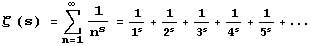The Riemann Hypothesis is currently the most famous unsolved problem in mathematics.  Like the Goldbach Conjecture (all positive even integers greater than two can be expressed as the sum of two primes), it seems true, but is very hard to prove.  I did some playing around with the Riemann Hypothesis, and I'm convinced it is true.  My observations follow.The Zeta Function
Euler showed that z(2) = p2/6 , and solved all the even integers up to z(26).  See the Riemann Zeta Function in the CRC Concise Encyclopedia of Mathematics for more information on this.  It is possible for the exponent s to be Complex Number (a + b I).  A root of a function is a value x such that f(x) = 0.

The Riemann Hypothesis :  all nontrivial roots of the Zeta function are of the form (1/2 + b I).

Mathematica can plot the Zeta function for complex values, so I plotted the absolute value of z(1/2 + b I) and z(1/3 + b I).|z(1/2 + b I)| for b = 0 to 85.  Note how often the function dips to zero.|z(1/3 + b I)| for b = 0 to 85.  Note how the function never dips to zero.
The first few zeroes of |z(1/2 + b I)| are at b = 14.1344725, 21.022040, 25.010858, 30.424876, 32.935062, and 37.586178.  Next, I tried some 3D plots, looking dead on at zero.  The plot of the function looked like this:Plot3D[Abs[Zeta[y+ x I]],{x,5,200},{y,.4,.6},PlotRange ->{0,.1}, PlotPoints ->200, ViewPoint->{200,.5,0}]
It seems like |z(a + b I)| is bounded away from zero when a doesn't equal 1/2.  Based on these plots and a few others, I'm fairly certain the Riemann Hypothesis is true.  The person who actually proves the hypothesis will be as famous as Andrew Wiles (he proved Fermat's Last Thereom).  By the analytic convergence theorem, we can get the slope: z'(x + q I) =.  Is it true that Re(z'(x + q I)) > 0 for x>1/2 and Re(z'(x + q I)) < 0 for x<1/2 ?

May 4 2002 -- The first ten billion zeroes are on the critical line, 1/2.